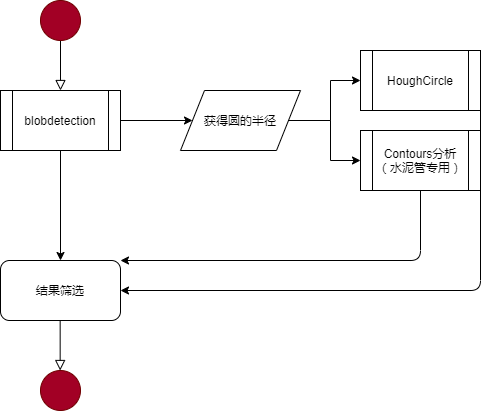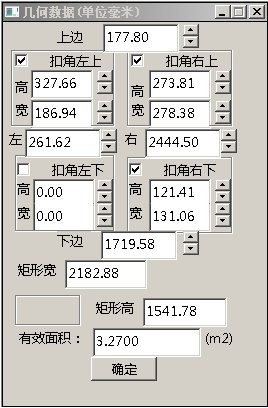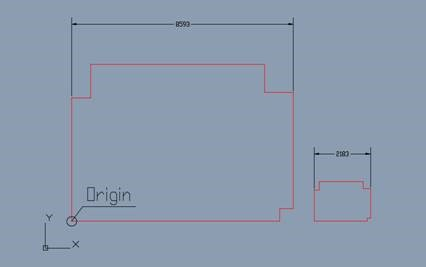# 找圆方法的总结和比较（三种主要识别方法的比较和融合）for (size_t i = 0; i < specialKeypoints.size(); i++)        {            bool isNear = true;            for (size_t j = 0; j < keypoints.size(); j++)            {                double dist = norm(specialKeypoints[i].pt - keypoints[j].pt);                isNear = (dist <= radius * 2.5f);                if (isNear)                {                    break;                }            }            if (isNear)            {                keypoints.push_back(specialKeypoints[i]);            }        }

        std::vector < KeyPoint > resultKeypoints;        for (size_t i = 0; i < keypoints.size(); i++)        {            bool isNew = true;            for (size_t j = 0; j < resultKeypoints.size(); j++)            {                double dist = norm(keypoints[i].pt - resultKeypoints[j].pt);                isNew  =  (dist >= keypoints[i].size/2 && dist >= resultKeypoints[j].size/2);                if (!isNew)                {                    break;                }            }            if (isNew && keypoints[i].size > radius)                resultKeypoints.push_back( keypoints[i]);        }

        // 基于Blob方法进行圆的寻找        SimpleBlobDetector::Params params;        params.filterByColor = false;        params.minThreshold = 0;        params.maxThreshold = 250;        vector<KeyPoint> keypoints;            cv::Ptr<cv::SimpleBlobDetector> detector = cv::SimpleBlobDetector::create(params);        detector->detect(srcNormal, keypoints);        //获得半径        std::vector<double> dists;        double radius;        for (size_t pointIdx = 0; pointIdx  < keypoints.size(); pointIdx++)        {            dists.push_back(keypoints[pointIdx].size);        }        std::sort(dists.begin(), dists.end());        radius = (dists[dists.size() / 2]) / 2.;        //基于HoughCircle方法进行圆的寻找        vector<KeyPoint> tmpKeypoints = findPipMethodHough(srcNormal, radius);        //基于轮廓方法，专门寻找“水泥管”        vector<KeyPoint> specialKeypoints = findConcretePip(srcNormal, radius);

 算法代码 注释讲解 SimpleBlobDetector::Params params;        params.filterByColor = false;        params.minThreshold = 0;        params.maxThreshold = 250;        vector keypoints;            cv::Ptr detector = cv::SimpleBlobDetector::create(params);        detector->detect(srcNormal, keypoints);        //获得半径        std::vector dists;        double radius;        for (size_t pointIdx = 0; pointIdx  < keypoints.size(); pointIdx++)        {            dists.push_back(keypoints[pointIdx].size);        }        std::sort(dists.begin(), dists.end());        radius = (dists[dists.size() / 2]) / 2.; 基于Blob方法进行圆的寻找，除了参数的定义有一些学问以外，基本上就是对自然图片进行基本处理，有个特点是然会radius Mat gray;    vector vecCenters;    vector vec3f_method_hough;    //处理彩色图片，进行Hough处理    if (src.channels() == 3 || src.channels() == 4)        cvtColor(src, gray, COLOR_BGR2GRAY);    else        gray = src.clone();    blur(gray, gray, cv::Size(3, 3));    HoughCircles(gray, vec3f_method_hough, HOUGH_GRADIENT, 2, p_radius*2, 100, 33, p_radius-2, p_radius + 2);    for (int i = 0; i < vec3f_method_hough.size(); i++)    {        Point center(cvRound(vec3f_method_hough[i]), cvRound(vec3f_method_hough[i]));        cv::KeyPoint kpt(center, (float)(p_radius * 2.0f));        vecCenters.push_back(kpt);    }    return vecCenters; 基于Hough方法进行圆的寻找，除了参数中radius是确定的以外，基本上就是通用的找圆算法 float f_area = CV_PI * p_radius * p_radius;//数学公式    vector vec_method_normal;//返回结果    Mat gray;    Mat tmp25;    Mat draw;    Mat srcClone = src.clone();    //局部阈值方法算法    cvtColor(src, gray, COLOR_BGR2GRAY);    blur(gray, gray, Size(3, 3)); //简单平滑    adaptiveThreshold(gray, tmp25, 255, ADAPTIVE_THRESH_MEAN_C, THRESH_BINARY,(int) p_radius * 2 + 1, 0.0);  //局部阈值        //形态学变换(膨胀)    Mat elementTest = getStructuringElement(MORPH_ELLIPSE, Size(5, 5));    morphologyEx(tmp25, tmp25, cv::MORPH_ERODE, elementTest);    //边界的钢管识别（边界填充）    rectangle(tmp25, cv::Rect(0, 0, tmp25.cols, tmp25.rows), Scalar(255, 255, 255), 1);    //轮廓分析    std::vector < std::vector > contours;    findContours(tmp25, contours, RETR_LIST, CHAIN_APPROX_NONE);    vector vecCenters;    int minArea = f_area * 0.5f;    int maxArea = 5000;    float minCircularity = 0.47f;    float maxCircularity = std::numeric_limits::max();    for (size_t contourIdx = 0; contourIdx < contours.size(); contourIdx++)    {        //筛选条件        Moments moms = moments(contours[contourIdx]);        //area        double area = moms.m00;        if (area < minArea || area >= maxArea)            continue;        //circularity        double perimeter = arcLength(contours[contourIdx], true);        double ratio = 4 * CV_PI * area / (perimeter * perimeter);        if (ratio < minCircularity || ratio >= maxCircularity)            continue;        Point2d center  = Point2d(moms.m10 / moms.m00, moms.m01 / moms.m00);        cv::KeyPoint kpt(center, (float)(p_radius * 2.0f));        vecCenters.push_back(kpt);            }    drawKeypoints(srcClone, vecCenters, draw, Scalar(0, 255, 0), DrawMatchesFlags::DRAW_RICH_KEYPOINTS);    return vecCenters; 虽然找不到几个目标，但是本函数是最复杂的（最长）的，在实现的过程中包含了圆度和面积的分析。特别是算法预处理这块，明显是有针对性地优化若干目标项目。

1、树叶测量

2、石材大板3、中药项目

4、答题卡项目

【想结合算法的设计实现，谈一些务虚的东西】

5.1、算法库的涉及范围广泛，需要进一步挖掘
OpenCV到底有多少算法，那些算法进行了优质实现？这些内容，如果不去进行具体研究，是很难得出明确结论的。这一次在findblob、hough的具体研究中，我发现OpenCV从算法本身、实现方法、实现细节，都是有很多值得深入研究的。

5.2、算法库影响广泛、代码规范，都是将来发展重要依托

5.3、Github的维护方式，是进一步“刻意练习”的重要依托
“可以练习”强调的是focus feed-back fix ，这在Github上面有非常好的体现，一定要寻找真正的专家，并且就普遍关心的问题进行深入的工作，这样才能进入能力提高“正循环”。

posted @ 2020-07-09 18:44  jsxyhelu  阅读(507)  评论(0编辑  收藏  举报